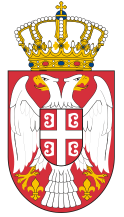Dokument se učitava

# Discrete MathematicsCourse code: 100507 | 6 ECTS credits

Basic information
Level of Studies: Undergraduate applied studies
Year of Study: 1
Semester: 2
Requirements:
Goal: Introduction to the mathematics of computer science, or the synthesis of various mathematical disciplines, such as mathematical logic, theory of sets, graph theory, combinatorics, and others.
Outcome: Students will be able to solve the problems of discrete structures that are the basis of the work of modern computer systems.
Contents of the course
Theoretical instruction:
1. Graphs.
2. Trees. Applications of trees in computing.
3. Relations at finite sets. Relation representation.
4. Relations of equivalence and order.
5. Introduction to Combinatorics.
6. First colloquium.
7. State account.
8. Boolean functions.
9. Predicate account.
10. Finite state automaton and regular grammar.
11. Connecting and minimizing automata.
12. Code Theory.
13. Second colloquium.
Practical instruction (Problem solving sessions/Lab work/Practical training):
1. Practical classes follow the program of lectures and take place through exercises in the computer laboratory, using the software package Maple.
Textbooks and References
1. A. Savić, S.Štrbac-Savić, Diskretna matematika i algoritmi, VIŠER, Beograd, 2015.
2. A. Savić, S.Štrbac-Savić, I.Stefanović, Diskretna matematika i algoritmi – priručnik za laboratorijske vežbe, VIŠER, Beograd, 2015.
Number of active classes (weekly)
Lectures: 3
Practical classes: 2
Other types of classes: 0
Grading (maximum number of points: 100)
Pre-exam obligations
Points
activities during lectures
0
activities on practial excersises
20
seminary work
0
colloquium
25
Final exam
Points
Written exam
25
Oral exam
30© 2019 School of Electrical and Computer Engineering of Applied Studies, Belgrade
Vojvode Stepe 283, office@viser.edu.rs, +381 11 2471 099